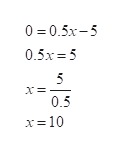I want to start a lemonade stand and determine how much lemonade I need to sell to break even each day. If I know I spend \$5 on supplies and sell the lemonade at \$.50 per glass determine the number of glasses I must sell to break even. After you find the number of glasses tell me what the x-intercept,y-intercept and slope represent in the function you determined.

Question

I want to start a lemonade stand and determine how much lemonade I need to sell to break even each day. If I know I spend \$5 on supplies and sell the lemonade at \$.50 per glass determine the number of glasses I must sell to break even. After you find the number of glasses tell me what the x-intercept,y-intercept and slope represent in the function you determined.

Step 1

Let x be the number of glasses of lemonades.

Given that, \$0.50 per glass is spent for lemonade each day.

The revenue by selling x glasses of lemonade is Revenue = 0.5x.

Given that, \$5 is spent on supplies.

The cost of the lemonade is cost = 5.

Step 2

The profit by selling x glasses of lemonade is

Step 3

The number of glasses that must sell to...help_outlineImage Transcriptionclose0 0.5x-5 0.5x 5 5 0.5 x=10 fullscreen

Want to see the full answer?

See Solution

Want to see this answer and more?

Our solutions are written by experts, many with advanced degrees, and available 24/7

See Solution
Tagged in

Algebra